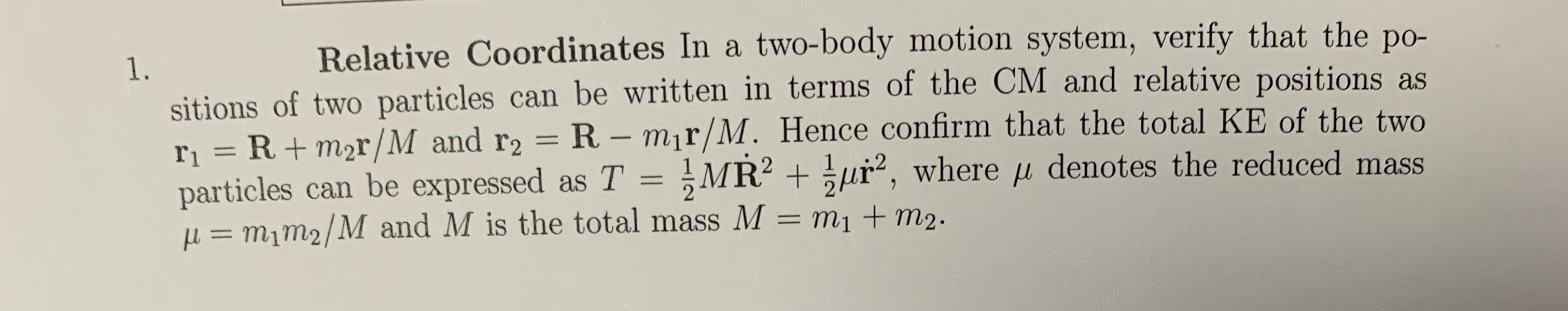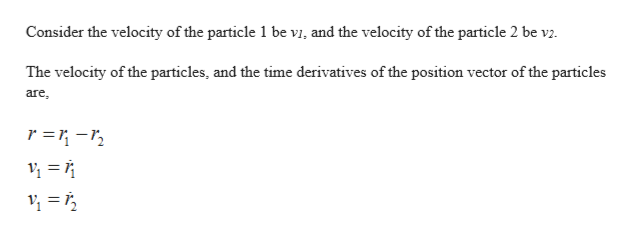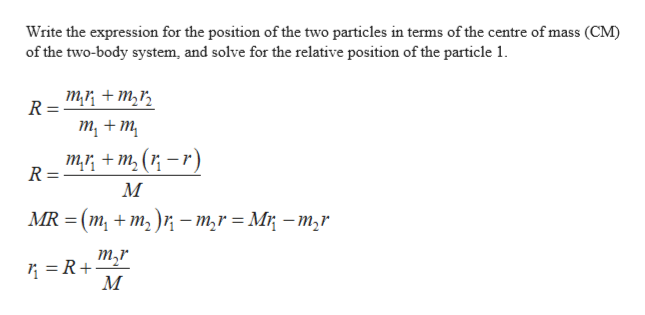# Relative Coordinates In a two-body motion system, verify that the po-1.sitions of two particles can be written in terms of the CM and relative positions asr1 = R+m2r/M and r2 = R - mir/M. Hence confirm that the total KE of the twoparticles can be expressed as T = MR2 ur, where u denotes the reduced mass= m1m2/M and M is the total mass M = m1 + m2.

Question
3 viewshelp_outlineImage TranscriptioncloseRelative Coordinates In a two-body motion system, verify that the po- 1. sitions of two particles can be written in terms of the CM and relative positions as r1 = R+m2r/M and r2 = R - mir/M. Hence confirm that the total KE of the two particles can be expressed as T = MR2 ur, where u denotes the reduced mass = m1m2/M and M is the total mass M = m1 + m2. fullscreen
check_circle

Step 1
Step 2help_outlineImage TranscriptioncloseConsider the velocity of the particle 1 be vi, and the velocity of the particle 2 be v2. The velocity of the particles, and the time derivatives of the position vector of the particles are r =1-r2 fullscreen
Step 3help_outlineImage TranscriptioncloseWrite the expression for the position of the two particles in terms of the centre of mass (CM) of the two-body system, and solve for the relative position of the particle 1 ти + т,, R т, + m, ти + m,(r-r) R М MR %3 (m, + m,)r — т,r %3D Mi — т,r т,r =R+ fullscreen

### Want to see the full answer?

See Solution

#### Want to see this answer and more?

Solutions are written by subject experts who are available 24/7. Questions are typically answered within 1 hour.*

See Solution
*Response times may vary by subject and question.
Tagged in

### Vectors and Scalars